# RS Aggarwal Class 6 Solutions Chapter 7 Decimals Ex 7D

## RS Aggarwal Class 6 Solutions Chapter 7 Decimals Ex 7D

These Solutions are part of RS Aggarwal Solutions Class 6. Here we have given RS Aggarwal Solutions Class 6 Chapter 7 Decimals Ex 7D.

Other Exercises

Question 1.
Solution:
27.86 from 53.74
= 53.74 – 27.86
= 25.88 Ans.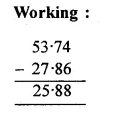Question 2.
Solution:
64.98 from 103.87
103.87 – 64.98
= 38.89 Ans.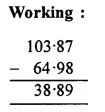Question 3.
Solution:
59.63 from 92.4
92.40 – 59.63
= 32.77 Ans.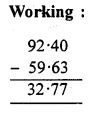Question 4.
Solution:
56.8 from 204
204.0 – 56.8
= 147.2 Ans.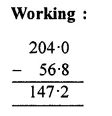Question 5.
Solution:
127.38 from 216.2
216.20 – 127.38
= 88.82 Ans.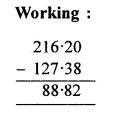Question 6.
Solution:
39.875 from 70.68
70.680 – 39.875
= 30.805 Ans.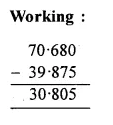Question 7.
Solution:
523.120 – 348.237
= 174.883 Ans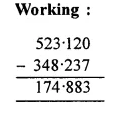Question 8.
Solution:
600.000 – 458.573
= 141.427 Ans.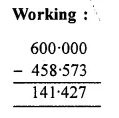Question 9.
Solution:
206.321 – 149.456
= 56.865 Ans.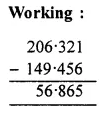Question 10.
Solution:
3.400 – 0.612
= 2.788 Ans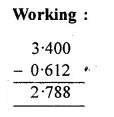Question 11.
Solution:
Converting them in like decimals
37.600 + 72.850 – 58.678 – 6.090
= (37.600 + 72.850) – (58.678 + 6.090)
= 110.450 – 64.768
= 45.682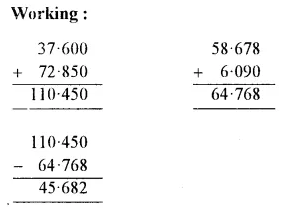Question 12.
Solution:
75.3 – 104.645 + 178.96 – 47.9
= 75.300 – 104.645 + 178.960 – 47.900
(Converting into like decimals)
= 75.300 + 178.960 – 104.645 – 47.900
= (75.300 + 178.960) – (104.645 + 47.900)
= 254.260 – 152.545
= 101.715 Ans.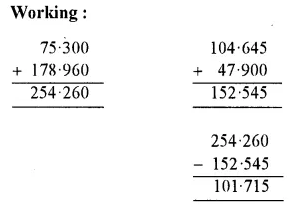Question 13.
Solution:
213.4 – 56.84 – 11.87 – 16.087
= 213.400 – 56.840 – 11.870 – 16.087
(Converting into like decimals)
= 213.400 – (56.840 + 11.870 + 16.087)
= 213.400 – 84.797
= 128.603 Ans.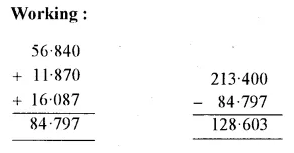Question 14.
Solution: 76.3 . 7.666 . 6.77
= 76.300 – 7.666 – 6.770
(Converting into like decimals)
= 76.300 – 14.436
= 61.864 Ans.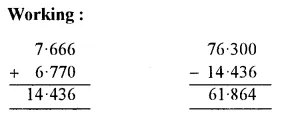Question 15.
Solution:
In order to get the required number, we have to subtract 74.5 from 91.
Required number = 91 – 74.5
= 91.0 – 74.5
= 16.5 Ans.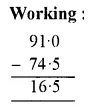Question 16.
Solution:
In order to get the required numbers, we have to subtract 0.862 from 7.3.
Required number = 7.3 – 0.862
= 7.300 – 0.862
= 6.438 Ans.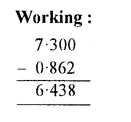Question 17.
Solution:
In order to get the required number, we have to subtract 23.754 from 50
Required number = 50 – 23.754
= 50.000 – 23.754
= 26.246 Ans.Question 18.
Solution:
In order to get the required number, we should subtract 27.84 from 84.5
Required number = 84.5 – 27.84
= 84.50 – 27.84
= 56.66 Ans.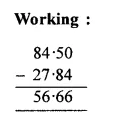Question 19.
Solution:
Weight of Neelam’s bag = 6 kg 80 g
Weight of Garima bag = 5 kg 265 g
Difference in their weights = 6 kg 80 g – 5 kg 265 g
= 6.080 kg – 5.265 kg
= 0.815 kg
= 815 g
Neelam’s bag is heavier by 815 g Ans.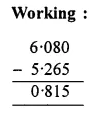Question 20.
Solution:
Cost of a notebook = Rs. 19.75
Cost of a pencil = Rs. 3 .85
Cost of a pen = Rs. 8.35
Total cost = Rs. 19.75 + Rs. 3.85 + Rs. 8.35
= Rs. 31.95
Amount given to the bookshop = Rs. 50
Balance amount to get back = Rs. 50.00 – Rs. 31.95
= Rs. 18.05 Ans.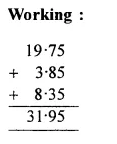Question 21.
Solution:
Weight of fruits = 5 kg 75 g .
Weight of vegetables = 3 kg 465 kg
Total weight of both = 5 kg 75 g + 3 kg 465 g
= 5.075 kg + 3.465 kg
= 8.540 kg
Gross weight of bag with these things = 9 kg
Net weight of bag = 9.000 – 8.540
= 0.460 kg
= 460 g Ans.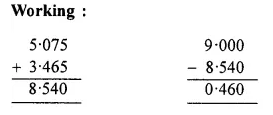Question 22.
Solution:
Total distance = 14 km
Distance covered by scooter = 10 km 65 m
Distance covered by bus = 3 km 75 m
Total distance covered by scooter and by bus = 10 km 65 m + 3 km 75 m
= 10.065 km + 3 075 m
= 13.140 km
Remaining distance covered by walking
= (14.000 – 13.140) km
= 0.860 km
= 860 m Ans.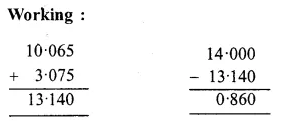Hope given RS Aggarwal Solutions Class 6 Chapter 7 Decimals Ex 7D are helpful to complete your math homework.

If you have any doubts, please comment below. Learn Insta try to provide online math tutoring for you.